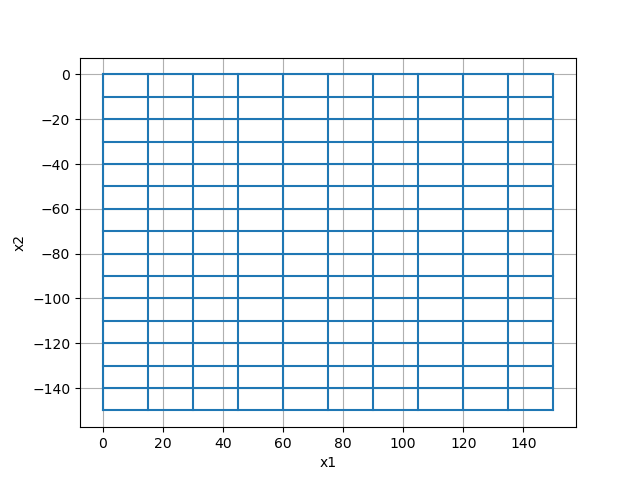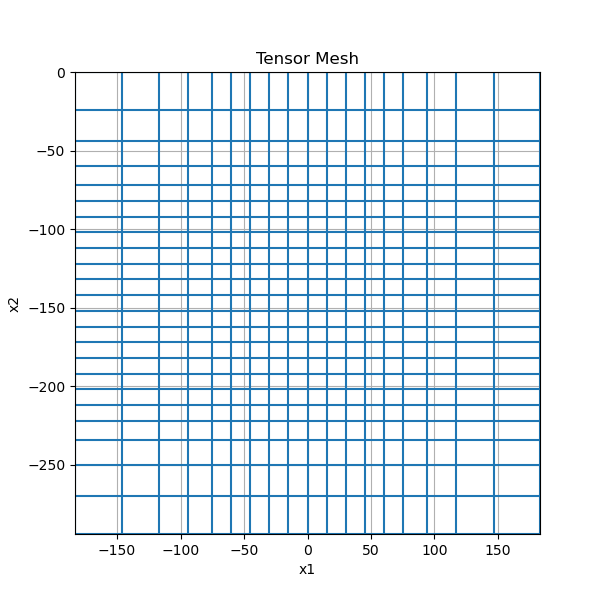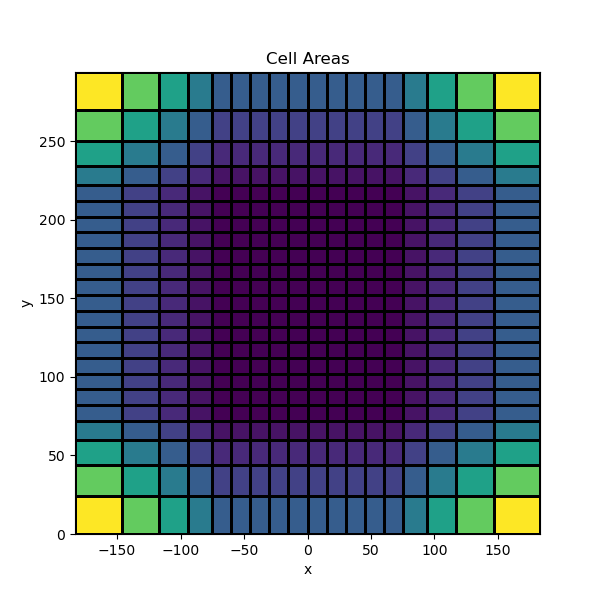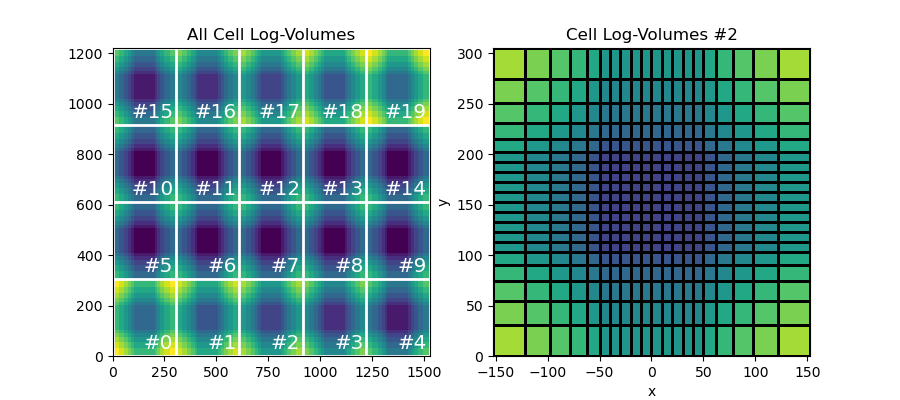# Tensor meshes#

Tensor meshes are the most basic class of meshes that can be created with discretize. They belong to the class (`TensorMesh`). Tensor meshes can be defined in 1, 2 or 3 dimensions. Here we demonstrate:

• How to create basic tensor meshes

• How to include padding cells

• How to plot tensor meshes

• How to extract properties from meshes

## Import Packages#

Here we import the packages required for this tutorial.

```from discretize import TensorMesh
import matplotlib.pyplot as plt
import numpy as np

# sphinx_gallery_thumbnail_number = 3
```

## Basic Example#

The easiest way to define a tensor mesh is to define the cell widths in x, y and z as 1D numpy arrays. And to provide the position of the bottom southwest corner of the mesh. We demonstrate this here for a 2D mesh (thus we do not need to consider the z-dimension).

```ncx = 10  # number of core mesh cells in x
ncy = 15  # number of core mesh cells in y
dx = 15  # base cell width x
dy = 10  # base cell width y
hx = dx * np.ones(ncx)
hy = dy * np.ones(ncy)

x0 = 0
y0 = -150

mesh = TensorMesh([hx, hy], x0=[x0, y0])

mesh.plot_grid()
``````<Axes: xlabel='x1', ylabel='x2'>
```

For practical purposes, the user may want to define a region where the cell widths are increasing/decreasing in size. For example, padding is often used to define a large domain while reducing the total number of mesh cells. Here we demonstrate how to create tensor meshes that have padding cells.

```ncx = 10  # number of core mesh cells in x
ncy = 15  # number of core mesh cells in y
dx = 15  # base cell width x
dy = 10  # base cell width y
exp_x = 1.25  # expansion rate of padding cells in x
exp_y = 1.25  # expansion rate of padding cells in y

# Use a list of tuples to define cell widths in each direction. Each tuple
# contains the cell width, number of cells and the expansion factor. A
# negative sign is used to indicate an interval where cells widths go
# from largest to smallest.

# We can use flags 'C', '0' and 'N' to shift the xyz position of the mesh
# relative to the origin
mesh = TensorMesh([hx, hy], x0="CN")

# We can apply the plot_grid method and output to a specified axes object
fig = plt.figure(figsize=(6, 6))
mesh.plot_grid(ax=ax)
ax.set_xbound(mesh.x0, mesh.x0 + np.sum(mesh.h))
ax.set_ybound(mesh.x0, mesh.x0 + np.sum(mesh.h))
ax.set_title("Tensor Mesh")
``````Text(0.5, 1.0, 'Tensor Mesh')
```

## Extracting Mesh Properties#

Once the mesh is created, you may want to extract certain properties. Here, we show some properties that can be extracted from 2D meshes.

```ncx = 10  # number of core mesh cells in x
ncy = 15  # number of core mesh cells in y
dx = 15  # base cell width x
dy = 10  # base cell width y
exp_x = 1.25  # expansion rate of padding cells in x
exp_y = 1.25  # expansion rate of padding cells in y

mesh = TensorMesh([hx, hy], x0="C0")

# The bottom west corner
x0 = mesh.x0

# The total number of cells
nC = mesh.nC

# An (nC, 2) array containing the cell-center locations
cc = mesh.gridCC

# A boolean array specifying which cells lie on the boundary
bInd = mesh.cell_boundary_indices

# Plot the cell areas (2D "volume")
s = mesh.cell_volumes

fig = plt.figure(figsize=(6, 6))
mesh.plot_image(s, grid=True, ax=ax)
ax.set_xbound(mesh.x0, mesh.x0 + np.sum(mesh.h))
ax.set_ybound(mesh.x0, mesh.x0 + np.sum(mesh.h))
ax.set_title("Cell Areas")
``````Text(0.5, 1.0, 'Cell Areas')
```

## 3D Example#

Here we show how the same approach can be used to create and extract properties from a 3D tensor mesh.

```nc = 10  # number of core mesh cells in x, y and z
dh = 10  # base cell width in x, y and z
exp = 1.25  # expansion rate of padding cells

mesh = TensorMesh([h, h, h], x0="C00")

# The bottom southwest corner
x0 = mesh.x0

# The total number of cells
nC = mesh.nC

# An (nC, 3) array containing the cell-center locations
cc = mesh.gridCC

# A boolean array specifying which cells lie on the boundary
bInd = mesh.cell_boundary_indices

# The cell volumes
v = mesh.cell_volumes

# Plot all cells volumes or plot cell volumes for a particular horizontal slice
fig = plt.figure(figsize=(9, 4))

mesh.plot_image(np.log10(v), grid=True, ax=ax1)
ax1.set_title("All Cell Log-Volumes")

cplot = mesh.plot_slice(np.log10(v), grid=True, ax=ax2, normal="Z", ind=2)
cplot.set_clim(np.min(np.log10(v)), np.max(np.log10(v)))
ax2.set_title("Cell Log-Volumes #2")
``````Text(0.5, 1.0, 'Cell Log-Volumes #2')
```

Total running time of the script: ( 0 minutes 0.393 seconds)

Gallery generated by Sphinx-Gallery